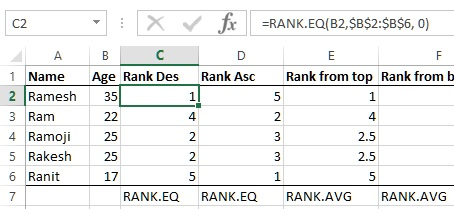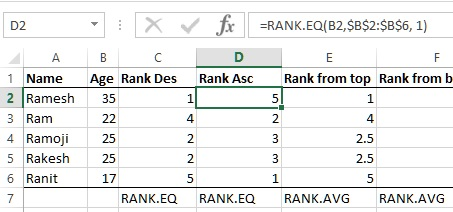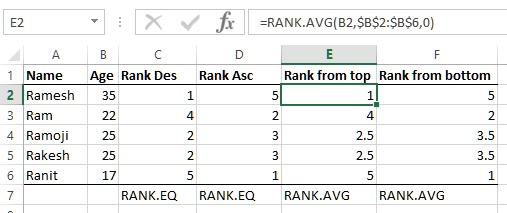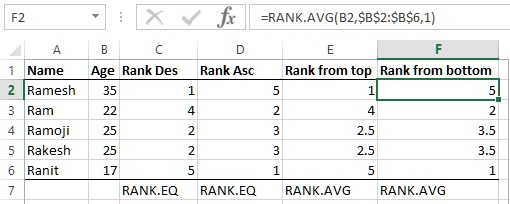# Ranking in Excel in Excel

##### How to return the rank of a number in list of numbers in Excel?

In last post, we learnt how to generate random number between two numbers in Excel. In this post, we shall learn how to rank a number in the list of numbers in Excel.

### RANK.EQ function

RANK.EQ function is equivalent to RANK function of older version of Excel. RANK.EQ function in Excel is used to return the rank of a number in a list of numbers; its size relative to other values in the list; if more than one value has the same rank, the top rank of that set of values is returned.

In below example, C1 cell has '=RANK.EQ(B2,\$B\$2:\$B\$6, 0)' formula where

• 1st paramter is the number to rank
• 2nd parameter is the list of cell reference from which the number to rank (\$B\$2 to \$B\$6)
• 3rd parameter is the order (0 = Ascending and 1 = Descending)
You must have noticed that the cell reference is given Absolute reference so that when the formula is copied to other cells, the cell range remains the same.D2 cell has the same formula however the 3rd parameter is 1 so this gives the rank in descending order.### RANK.AVG function

RANK.AVG function in Excel is used to return the rank of a number in the list of numbers; its size relative to other values in the list; if more than one value has the same rank, the average rank is returned.

In below example, E2 cell has '=RANK.AVG(B2,\$B\$2:\$B\$6,0)' formula where

• 1st paramter is the number to rank
• 2nd parameter is the list of cell reference from which the number to rank (\$B\$2 to \$B\$6)
• 3rd parameter is the order (0 = Ascending and 1 = Descending)

Notice the E4 and E5 cell whose value is 2.5 as the RANK of B4 and B5 cells are same (Assuming E4 rank as 2 and E5 rank as 3 so the average is (2+3/2) = 2.5.When the 3rd parameter is 1, the ranking is done in descending order as shown in F2 cell.Hope this helps in understanding RANK function in Excel. If this helps, do subscribe and share to your friends and colleagues.

Views: 2424 | Post Order: 39